# 15. A final example.

Example: Consider the differential equationWe get the outer approximation by putting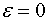and solving the equation with the outer boundary condition:that is,We now look for the inner approximation. PutThe equation is then transformed to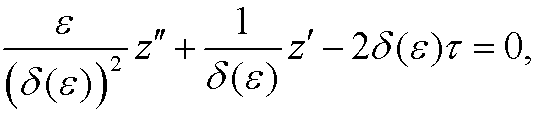where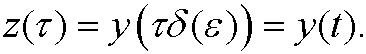with the other (is supposed to be small):

 Case1):Case 2):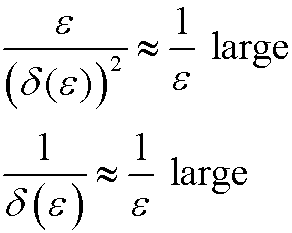We see that in Case 2, the remaining coefficient is much smaller than the other two. We therefore chooseand make the change of variablesOur equation then becomesWe get the inner approximation when we putand solve the equation: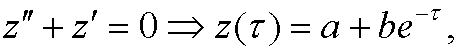which in the original variables isThe condition y(0)=1 then givesLet us match these approximations. Introduce the intermediate variableThe matching conditionthen implies thatthat is,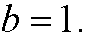Our inner approximation finally becomesWe get a unit approximation yu by adding the inner and outer approximations and subtracting their common limit in the overlapping region